iSoul In the beginning is reality.

# Transformations for one or two directions

A bidirectional transformation applies to all observers, and so must work for any direction, including observer and observed with the roles switched. A physics for all observers should be bidirectional if possible. This works for mechanics but for thermodynamics entropy is inherently directional.

The Galilean transformation is for one direction with no characteristic (modal) rate. As I showed here, there is no consistent way to make this bidirectional. The Lorentz transformation is bidirectional. That is why the directional transformations are multiplied together in the derivation.

The Galilean transformation for one direction with spatial axis r and temporal axis t is:

Speed: r′ = r – vt and t′ = t,

Pace: t′ = t – ru and r′ = r.

A similar transformation for one direction with a characteristic rate c is:

Speed: r′ = rtv = r (1 – v/c) and

t′ = t (1 – v/c) = t – (r/c) (v/c) = t – r (v/).

Pace: t′ = t – ru = t – r/v = t (1 – c/v) and

r′ = r (1 – u/b) = r (1 – c/v) = r − ct (c/v) = rt (/v).

The Lorentz transformation (bidirectional with characteristic rate) is:

r′ = γ (r – tv) and t′ = γ (t – rv/) with γ = (1 – v²/c²)–1/2,

which applies only if |v| < |c|. The symmetric version is:

r′/c = γ (r/c – t (v/c)) = γt (1 – v/c), and

ct′ = γ (ct – r (v/c)) = γr (1 – v/c).

In the Lorentz transformation time is an independent variable; if space is independent:

r′ = γ (rc2 t/v) and t′ = γ (t − r/v) with γ = (1 − c2/v2)–1/2,

which applies only if |v| > |c|. The symmetric version is:

r′/c = γ (r/c – t (c/v)) and ct′ = γ (ct – r (c/v)).

# Travel time and temporal displacement

There’s a basic distinction between the travel distance (or flight length) and the displacement. There should be a corresponding distinction between the travel time (or flight time) and the temporal displacement – which I’ll call the dischronment (dis-time-ment vs. dis-place-ment).

The travel time is the total duration of the trip, and the travel distance is the total distance traveled (odometer length). The displacement is the result of the change in position, the resultant position vector. Similarly, the dischronment is the result of the change of the point in time, the resultant time position vector.

A round-trip begins and ends at the same place; its displacement is zero. However, the place is not exactly the same because changes are always occurring – you can’t step in the same river twice (Heraclitus). Temporally, a round-trip begins at a certain point in time – an event – and returns to the ‘same’ event so that its time displacement is zero. It’s not exactly the same event but a parallel event such as opening the door to the same home.

Sometimes in my career when I went on official travel, I would take a personal side trip at my own expense. In order to make the official travel voucher as straightforward as possible, I would begin and end my side trip at the same time of day on a subsequent day. For example, if my official business was complete at 5:00 pm Thursday, instead of taking a flight home I would switch to personal time and go off a few days on my own. Then at 5:00 pm on, say, Saturday, I would switch back to official time and fly home on official travel. The time displacement of the side trip was zero, which made it easy to remove the personal time from the official per diem allowance.

Speed is the ratio of the distance traveled to the travel time, that is, distance over duration. Velocity is the ratio of the change in position – the displacement – to the travel time. Lenticity should be defined analogously as the ratio of the change in temporal position – the dischronment – to the distance traveled.

The dependent variable in velocity is the displacement. Similarly, the dependent variable in lenticity is the dischronment. The independent variable in velocity is the travel time for a single motion but for multiple motions the independent variable is the magnitude of the dischronment, not the total travel time.

Similarly, the independent variable in lenticity is the travel distance for a single motion but for multiple motions the independent variable is the magnitude of the displacement, not the total distance traveled. Thus the resultant of several velocities or lenticities is their vector sum.

If someone travels 30 km North in 4 hr, then 40 km East in 3 hr, their displacement is 50 km Northeast at 53 degrees from the North and their dischronment is 5 hr Northeast in a course 37 degrees clockwise from the North. Their velocities are 30 km/4 hr = 7.5 km/hr North and 40 km/3 hr = 13.3 km/hr East.

The trip took 3 + 4 = 7 hr. So is their resultant velocity 50 km/7 hr? Or is the resultant velocity the displacement divided by the magnitude of the dischronment, 50 km/5 hr = 10 km/hr? It is the latter. So the dischronment is really not new.

Similarly, their lenticities are 4/30 = 8 min/km North and 3/40 = 4.5 min/km East. The distance traveled is 30 + 40 = 70 km. Is their resultant lenticity 5 hr/70 km? No, it’s the dischronment divided by the magnitude of the displacement, 5 hr/50km = 6 min/km.

One might define the effective velocity of a trip as the displacement divided by the total travel time. And the effective lenticity of a trip might be defined as the dischronment divided by the total travel distance. But these are different from the resultant vectors of velocity and lenticity.

# Time scale maps

Maps of travel times have various names, e.g., time scale maps, isochrone maps, etc. Often a geographic map forms the background so that travel time is superimposed on distance traveled. Occasionally, the time scale replaces the distance scale and the map looks distorted from a geographic perspective, but is correct from a travel time perspective. For example, here is the Washington, DC, Metro (subway) map in two forms, the left in geographic form and the right in time scale form: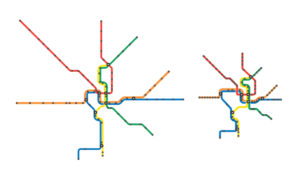Here is a map of Europe, on the left in geographic form, then representing rail travel times, and on the right, high speed rail travel times:Next is an outline of travel times by train from Paris superimposed on an outline of France: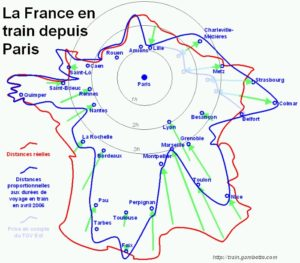Just as maps of distance show space in two dimensions, so maps of travel time show time in two dimensions.

# A new geometry for space and time

This blog has described how as the distances between places cover three dimensions of space, so the durations between events cover three dimensions of time. One way of looking at this is as a map with the distance and duration given between places, such as this from the Interstate Drive Times and Distances:There are two numbers for each leg or link in the map; one number in red for the distance in miles and another in blue for the time in hours and minutes. The durations are not proportional to the distance, which reflects the differing local conditions and topography.

It is common to adopt a speed that represents the typical speed for the mode of travel, which I’m calling the modal speed (or rate). This serves various purposes, one of which is for pre-trip planning to estimate the travel time to a destination. It can also serve as the conversion of distance and duration for the mode, much as the speed of light serves for relativity.

Divergence from the modal rate may be represented through a third dimension (z), similar to a topographic map. The modal rate then would be represented by a flat topography. A link with greater distance than the modal one would be like a positive z coordinate, and a link with less than the modal one would be like a negative z coordinate. The resultant link would be determined by the Pythagorean theorem for positive values of z, and by a hyperbolic version (as if z were imaginary) for negative values of z.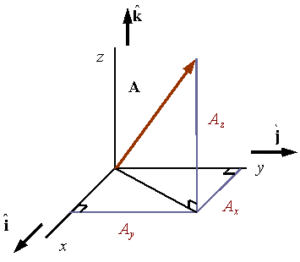That is, |A|² = x² + y² + z² if z > 0 (Pythagorean), and |A|² = x² + y² – z² if z < 0 (hyperbolic). For positive values of z, the magnitude of the resultant link is greater than unity, that is, greater than the modal rate. But for negative values of z, the magnitude of the resultant link is less than unity, that is, less than the modal rate.

The result may be mapped like a contour or isoline map, except that negative values have a hyperbolic geometry. The simplest way to see this is to take Δt = 1 and Δr = > 1, which uses the Pythagorean theorem. If either the time interval changes to Δt < 1 or the space interval is reduced to Δr < 1, the hyperbolic version is used for the link.

# Why time is three dimensional

The case for 3D time is very simple: space is based on the measurement of distance and time is based on the measurement of duration. As the distances between places cover three dimensions of space, so the durations between events cover three dimensions of time. As distance may be measured going from or toward a space-time point, so duration may be measured going from or toward a point in time-space.

In everyday life we speak of how far away a place is as a length of space or a length of time for a mode of travel. For example: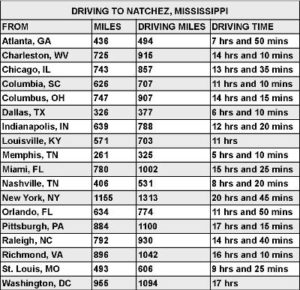Can this informal way of speaking be formalized in the language of physics? Yes.

The difference between space and time is that distance is measured asynchronously, whereas duration is measured synchronously. One must synchronize a motion with a standard motion – called a clock – in order to measure time. A clock measures one dimension of time in one direction (or without regard to direction). A rod or rule is used asynchronously to measure a motion before, during, or after the fact – in one direction.

The context requires a mode of travel in which there is a modal rate of travel that can be used to convert space and time into one another. This is commonly done in physics with the speed of light. Length can be defined in terms of the distance traveled for a specified time at the speed of light (and the standard meter is defined that way, see here). Time can be defined in terms of the travel time for a specified distance at the speed of light. The independence of this modal rate from any particular speed of travel leads to relativity theory.

Direction is determined by the relation of a motion to a spatio-temporal point, which is either a point from which the motion comes or toward which the motion is going. For example, magnetic north is a point on earth toward which compasses point. This point moves slowly, so for accuracy surveyors must specify the year a measurement was taken.

As space has direction in three dimensions, so does time. For more about 3D time, there is a list of posts here.

# Necessary and possible dimensions

In everyday life 1D space and 1D time are typically used. We are concerned with how far away something is (travel length), how long will it take to get there (travel time), what the speed is (apart from direction). Unless we are doing something in which size or direction are important, 2D is all we need.

Only two dimensions are necessary: 1D space and 1D time. Anything beyond this is possible but not necessary – up to a limit of 3D space and 3D time. Thus it is possible to define, measure, and adopt conventions in which there is exactly 3D space and 1D time. But it is also possible to define, measure, and adopt conventions in which there is exactly 1D space and 3D time. And it may be possible to define, measure, and adopt conventions in which there is exactly 3D space and 3D time.

So it is no objection to 3D time that it can be defined to be 1D. It is also no objection to 1D space that it can be defined to be 3D. Other conventions are possible. And a 3D space and 1D time convention is symmetric with a 1D space and 3D time convention.

# Time conventions

The natural concept of time is solar time, which is based on the Sun’s position in the sky. But local solar time or local mean time varies by longitude. With the spread of railroads in the 19th century, there was a need for time zones to standardize time and simplify east and west travel.

The 1884 International Meridian Conference adopted a universal day beginning at Greenwich midnight, but did not specify any local times or time zones. Over the years since then each country has determined their own time zones, usually offset by one hour from Greenwich mean time (GMT), but in some cases offset by one-half or one-quarter hour.

If one travels far enough in an east or west direction, a time zone will be crossed. In general this differs from travel north or south. A traveler going west is said to gain time as the clock is turned back, and a traveler going east is said to lose time as the clock is moved forward. The difference in time between east-west travel and north-south travel shows that time is capable of having two dimensions.

Coordinated universal time (UTC) is based on International Atomic Time, which is a weighted average of the time kept by over 400 atomic clocks in over 50 national laboratories worldwide. This time convention does not vary by location.

# Paceometer

We’ve all seen a vehicle speedometer, scaled in km/h or mph like this: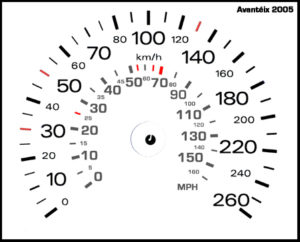A paceometer is made by adding another scale, as with the outer scale of minutes per 10 miles below (image source here):Fitness apps on smartphones can display distance traveled, travel time, and pace. In the article Pace yourself: Improving time-saving judgments when increasing activity speed, the authors conclude:

In this paper, we tried to highlight a general cognitive phenomenon in which people misestimate time-savings when increasing speed and suggested that this bias is due to the commonly used speed measures that extenuate people’s inability to recognize the curvilinear relationship between these speed measures and the reduction of activity time. We offer a simple remedy for this bias: converting speed values to pace data, which simplifies the cognitive task of judging time-savings because the data conforms to people’s lay perceptions. We believe that this simple solution can be applied to other contexts in which individuals’ judgments might be biased.

Here’s a table to convert miles per hour to minutes per 10 miles, or kilometers per hour to minutes per 10 kilometers – note it’s the same conversion, relatively speaking:

 Speed Pace mph min/10mi kph min/10km 0 0 5 120 10 60 15 40 20 30 25 24 30 20 35 17 40 15 45 13 50 12 55 11 60 10 65 9.2 70 8.6 75 8.0 80 7.5 85 7.1 90 6.7 95 6.3 100 6.0 105 5.7 110 5.5 115 5.2 120 5.0

# Lorentz transformation for 3D duration

It is worth returning to my post on Lorentz and Dual Lorentz transformations in order to make the point that the Lorentz transformation is sufficient for 3D time. Superluminal speeds are not required for 3D time, contrary to what others have said, such as here.

What is required for 3D duration (with 1D stance) is the use of measures relating measured changes in duration to independent changes in length. Pace, legerity, and lentation are needed instead of speed, velocity, and acceleration. See glossary above for definitions of terms.

The conception of length space is then simplified to a one-dimensional distance from some conventional origin point, the stance. This conception of stance is analogous to the common conception of time, as something that flows on independently of us. That is what it means to be an independent variable: it’s out of our control.

# Synchrony conventions

Reichenbach and Grünbaum noted that “the relation of simultaneity within each inertial reference frame contains an ineradicable element of convention which reveals itself in our ability to select (within certain limits) the value to be assigned to the one-way speed of light in that inertial frame.” (John A. Winnie, “Special Relativity without One-Way Velocity Assumptions: Part I,” Philosophy of Science, Vol. 37, No. 1, Mar., 1970, p. 81.)

Because the speed of light is measured as a round-trip speed, the one-way speed of light is unknown. It is a convention. This thesis is called the conventionality of simultaneity. Winnie writes (p. 82):

At time t1, by the clock at A, a light beam is sent to B and reflected back to A. Suppose that the return beam arrives at A at time t3 by the A-clock. The question now arises: at what time t2 (by the A-clock) did the beam arrive at B? Were we to “postulate” that the back and forth travel-times of the light beam were equal, clearly the answer is:

(1-1 ) t2 = t1 + (t3 – t1)/2

But should we fail to postulate the equality of the back-and-forth travel times, the best that we could do in this case would be to maintain that t2 is some timepoint between t1 and t3. Thus (using Reichenbach’s notation) we have the claim:

(1-2) t2 = t1 + ε(t3 – t1), (0 < ε < 1).

The simplest convention is one in which ε = ½, which is what Einstein suggested, by synchronizing clocks this way and slowly transporting them to other locations. It is called Einstein synchronization or synchrony convention (ESC). That way, the speed of light has the same value going and coming; it is a constant, c.

What would Galileo do? To preserve the Galilean transformation as much as possible, the speed of light either outgoing or incoming could be made effectively infinite. That would require a value of ε that is 0 (infinite speed outgoing) or 1 (infinite speed incoming). Such a Galilean synchrony convention (GSC) would need the speed of light in the other direction to equal c/2, that is, half of the ESC speed of light. That is because the harmonic mean of infinity and c/2 equals c (sequential speeds are averaged by the harmonic mean, not the arithmetic mean). Jason Lisle proposed such an anisotropic synchrony convention (ASC).

Synchrony is also required for those who use the diurnal movement of the sun and stars to tell time, i.e., the apparent or mean solar time. This applies to travel on or near the surface of the earth. The round trip time should not be affected by latitude or longitude, but without a correction for longitude, the travel time east or west would not match the return trip because of the direction of the sun’s motion. If one did not correct for latitude, travel north or south could also not match the return trip. It would be possible for a airplane to fly west with the sun or stars in the same position, so that no time elapsed in this sense.